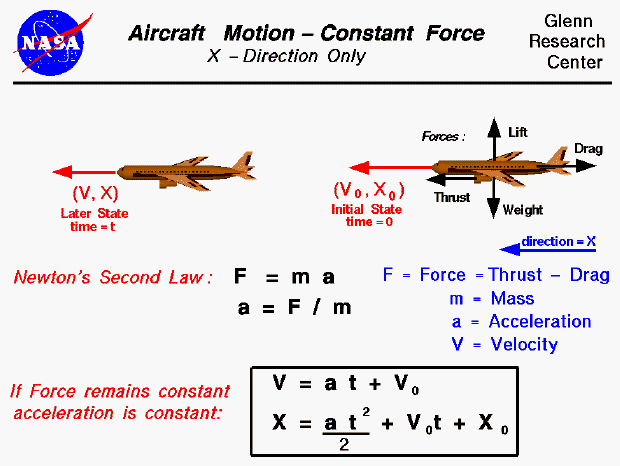An interactive Java applet that demonstrates the information found on this slide is also available at this web site. The applet presents problems that you must solve by using the equations of motion.

As discussed on the airplane cruise slide, an airplane can sustain a constant speed and level flight in which the weight is balanced by the lift, and the drag is balanced by the thrust. However, if the forces become unbalanced, the aircraft will move in the direction of the greater force. We can compute the acceleration of the aircraft from Newton's Second Law of Motion. If the mass of the aircraft remains a constant we can use the familiar form of the equation to solve for the acceleration (a)

F = m * a

a = F / m

We first have to determine the mass (m) of the aircraft from the weight. The force (F) will be the difference between the opposing forces (lift minus weight or thrust minus drag).

If the force also remains constant, the basic equations of motion can be solved. For a constant force and constant mass, the acceleration remains constant. The velocity (V) at any time (t) is the acceleration times the time, plus the initial velocity (Vo).

V = a * t + Vo

Similarly, the location (X) at any time (t) is given by 1/2 the acceleration times the time squared, plus the initial location (Xo), plus the initial velocity times the time.

X = .5 * a * t^2 + Vo * t + Xo

Note that these equations can be used only if the mass and the force (and the acceleration) are constant. The mass of an aircraft remains fairly constant during cruise since the only loss is for the fuel which is consumed. Fuel mass is normally a small percentage of the mass of an aircraft. However for aircraft, the lift and drag forces are themselves functions of the square of the velocity. So we can assume a constant force for only a very small amount of time. To solve the actual equations of motion for an aircraft, we must use calculus and integrate the equations of motion. The integration can be performed analytically or numerically.

Guided Tours

Beginner's Guide to Aerodynamics
Beginner's Guide to Propulsion
Beginner's Guide to Model Rockets
Beginner's Guide to Kites
Beginner's Guide to Aeronautics